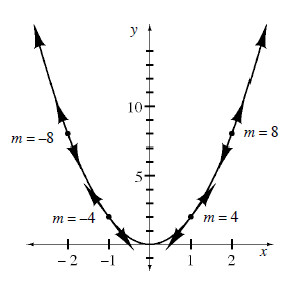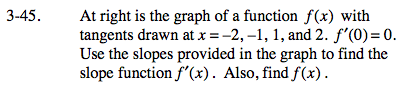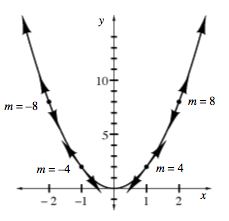### Home > CALC > Chapter 3 > Lesson 3.2.1 > Problem3-45

3-45.
1. Below is the graph of a function f(x) with tangents drawn at x = −2, −1, 1, and 2. f ′(0) = 0. Use the slopes provided in the graph to find the slope function f ′(x). Also, find f(x). Homework Help ✎First make a prediction about the shape of the slope graph. The original graph is a parabola. What will the slope graph look like?

Next, make a table showing x-values and their corresponding slopes. Be sure to include that when x = 0, the slope is 0 (after all, look at the horizontal tangent line).

Use your table to find an equation of the slope function.